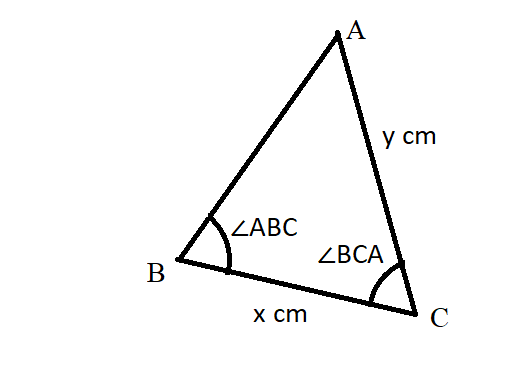Ask question

# The measure of the complement of an angle is three times the measure of the angle. What is the measure of each angle?# The measure of the complement of an angle is three times the measure of the angle. What is the measure of each angle?

Question
Non-right triangles and trigonometryasked 2020-11-30
The measure of the complement of an angle is three times the measure of the angle. What is the measure of each angle?

## Answers (1)2020-12-01
If x is the measure of an angle, then 90−x is the measure of its complement because complementary angles have an angle sum of 90°.
The measure of the complement of an angle is three times the measure of the angle so we write:
90−x=3(x)
Solve for x:
90−x=3x
90=4x
22.5=x
So, the measures of the angles are:
22.5° and 67.5°

### Relevant Questionsasked 2021-02-02

The measure of the supplement of an angle is $$\displaystyle{40}^{{\circ}}$$ more than three times the measure of the original angle. Find the measure of the angles. Instructions: Use the statement: " Let the original angle be x " to begin modeling the working of this question.

a) Write algebraic expression in terms of x for the following:

I) $$40^{\circ}$$ more than three times the measure of the original angle

II) The measure of the Supplement angle in terms of the original angle, x

b) Write an algebraic equation in x equating I) and II) in a)

c) Hence solve the algebraic equation in

b) and find the measure of the angles.asked 2021-03-06
If the measure of an angle is 23°17′18′′, what is the measure of its supplement?asked 2020-11-08
In triangle ABC, angle B is five times as large as angle A. The measure of angle C is 2° less than that of angle A. Find the measures of the angles. (Hint: The sum of the angle measures is 180°.)asked 2020-11-06
In $$\displaystyle△{P}{Q}{R},{m}∠{P}={46}{x}$$ and $$\displaystyle{m}∠{R}={7}{x}$$,
a. Write and solve an equation to find the measure of each angle.
b. Classify the triangle based on its angles.asked 2020-11-24
[Triangle] Determine the measure of each interior angle of △MAN and classify the triangle. m m /trnglMAN is a(n)_______________ triangle.asked 2021-02-06
The measure of an angle is 6 less than 5 times its complement. What is the measure of the complement?asked 2021-02-05
Two angles are supplementary. One of the is 18 more than 6 times the other. How large is the larger angle?asked 2020-12-27
Suppose that $$\displaystyle{m}\angle{B}{C}{A}={70}^{\circ}$$, and x=33 cm and y = 47 cm. What is the degree measure of $$\displaystyle\angle{A}{B}{C}$$?asked 2021-04-13
As depicted in the applet, Albertine finds herself in a very odd contraption. She sits in a reclining chair, in front of a large, compressed spring. The spring is compressed 5.00 m from its equilibrium position, and a glass sits 19.8m from her outstretched foot.
a)Assuming that Albertine's mass is 60.0kg , what is $$\displaystyle\mu_{{k}}$$, the coefficient of kinetic friction between the chair and the waxed floor? Use $$\displaystyle{g}={9.80}\frac{{m}}{{s}^{{2}}}$$ for the magnitude of the acceleration due to gravity. Assume that the value of k found in Part A has three significant figures. Note that if you did not assume that k has three significant figures, it would be impossible to get three significant figures for $$\displaystyle\mu_{{k}}$$, since the length scale along the bottom of the applet does not allow you to measure distances to that accuracy with different values of k.asked 2020-12-06
if $$\displaystyle\angle{c}=\angle{b}{\quad\text{and}\quad}\angle{a}={4}\angle{b}$$, find the value of each angle.
...